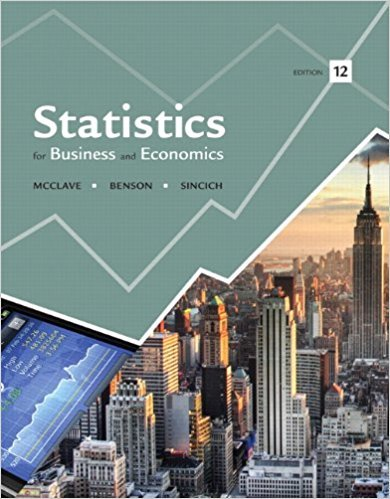×
Get Full Access to Statistics For Business And Economics - 12 Edition - Chapter 8 - Problem 46e
Get Full Access to Statistics For Business And Economics - 12 Edition - Chapter 8 - Problem 46e

×

# Construct a 95% confidence interval for (p1 - p2) in eachISBN: 9780321826237 51

## Solution for problem 46E Chapter 8

Statistics for Business and Economics | 12th Edition

• Textbook Solutions
• 2901 Step-by-step solutions solved by professors and subject experts
• Get 24/7 help from StudySoup virtual teaching assistantsStatistics for Business and Economics | 12th Edition

4 5 1 276 Reviews
31
0
Problem 46E

Problem 46E

Construct a 95% confidence interval for (p1 - p2) in each of the following situations:

a. n1 = 400, 1 = .65; n2 = 400, 2 = .58

b. n1 = 180, 1 = .31; n2 = 250, 2 = .25

c. n1 = 100, 1 = .46; n2 = 120, 2 = .61

Step-by-Step Solution:
Step 1 of 3

Chapter 8: bivariate correlational research - When you see two things that are related, and you think are related, but actually have nothing to do with one another To be causal - Covariance - Internal validity - Temporal precedence Correlational strength - As you get a measurement change by this much on this chart or whatever - You get the same ratio change on the other chart for the other variable - This means that they are changing at the same rate o Either moving at the same amount in the same direction [perfect correlation o Or moving at the same amount in the opposite direction [perfect correlation - When they don’t move at the same rate; imperfect correlation – this is what r

Step 2 of 3

Step 3 of 3

##### ISBN: 9780321826237

Unlock Textbook Solution